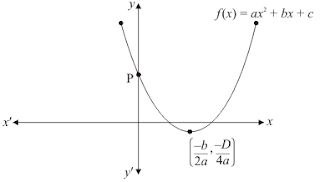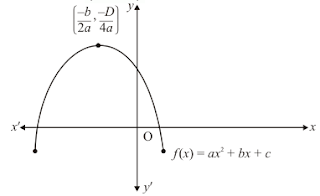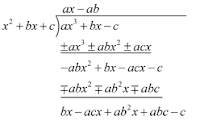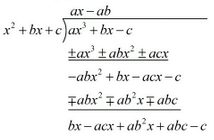>

## Chapter 2 Polynomials R.D. Sharma Solutions for Class 10th Math MCQ's

Multiple Choice Questions

1. If α, β are the zeros of the polynomial f(x) = x2 + x + 1 , then 1/α + 1/ β =
(a) 1
(b) -1
(c) 0
(d) None of these

Solution

Since α and β are the zeros of the quadratic polynomial f(x) = x2 + x + 1

α + β = - coefficient of x/coefficient of x2
= -1/1 = 1
α × β = constant term / coefficient of x2
= 1/1 = 1
We have
1/α + 1/β =
= β+α/ αβ
= -1/1
= -1
The value of 1/α + 1/β is -1
Hence , the correct choice is b .

2. If α, β are the zeros  of the polynomial p(x) = 4x2 + 3x + 7, then 1/α + 1/β is equal to

(a) 7/3
(b) – 7/3
(c) 3/7
(d) -3/7

Solution

Since α and β are the zeros of the quadratic polynomial p(x) = 4x2 + 3x + 7
α + β = -coefficient of x / coefficient of x2
= -3/4
= αβ = Constant term / Coefficient of x2
= 7/4
We have3. If one zero of the polynomial f(x) = (k2 + 4)x2 + 13x + 4k is reciprocal of the other, then k =
(a) 2
(b) -2
(c) 1
(d) -1

Solution

We are given f(x) = (k2 + 4)x2 13x + 4k then
α + β = -Coefficient of x/ Coefficient of x2
= -13/k2 + 4
α ×  β = Constant term / Coefficient of x2
= 4k/k2 + 4
One root of the polynomial is reciprocal of the other. Then , we have
α ×  β = 1
= 4k/k2+4
= 1
= k2 – 4k + 4 = 0
= (k-2)2 = 0
= k = 2
Hence the correct choice is a .

4. If the sum of the zeros of the polynomial f(x) = 2x3 − 3kx2 + 4x − 5 is 6, then the value of k is

(a) 2
(b) 4
(c) −2
(d) −4
Solution

Let α, β be the zeros of the polynomial f(x) = 2x3 – 3kx2 + 4x -5 and we are that α + β + γ = 6 .
Then,
α + β + γ = -Coefficient of x/Coefficient of x2
= - (-3k)/2 = 3k/2
It is given that
α + β + γ = 6
Substituting α + β + γ = 3k/2 , we get
+3k/2 = 6
+ 3k = 6 × 2
+3k = 12
k = 12/+3
k = +4
The value of k is 4 .
Hence, the correct alternative is b .

5. If α and  β are the zeros of the polynomial f(x) = x2 + px + q, then  a polynomial having  1/α and 1/ β is its zero is
(a) x2 + qx + p
(b) x2 – px + q
(c) qx2 + px + 1
(d) px2 + qx + 1

Solution

Let α and  β are the zeros of the polynomial f(x) = x2 + px + q . Then ,
α + β = -Coefficient of x/ Coefficient of x2
= -p/1
= -p
And
αβ = Constant term/Coefficient of x2
= q/1
= q
Let S and R denote respectively the sum and the product of the zeros of a polynomial
Whose zeros are 1/α and 1/β . then
S = 1/α + 1/β
= α+ β/ αβ
= -p/q
R =  1/α × 1/β
= 1/ αβ
= 1/q
Hence, the required polynomial g(x) whose sum and product of zeros are S and R is given by
x2  - Sx + R = 0
x2 + P/q x + 1/q = 0
qx2 + Px + 1/q = 0
qx2 + Px + 1
So g(x) = qx2 + Px + 1
Hence , the correct choice is c .

6. If α,β are the zeros of polynomial f(x) = x2 – p(x+1) – c , then (α + 1) (β + 1) =
(a) c – 1
(b) 1 – c
(c) c
(d) 1 + c

Solution

Since, α and β are the zeros of quadratic polynomial
f(x) = x2 – p(x+1)-c
= x2
α + β = -Coefficient of x/Coefficient of x2
= -(-p/1)
= p
α×β = Constant term/Coefficient of x2
= -p-c/1
= -p-c
We have
(α +1)( β+1)
αβ + β + α + 1
= αβ + (α+β) + 1
= - p - c + (p) + 1
= - p - c + (p) + 1
= -c + 1
= 1 –c
The value of (α +1)( β+1) is 1 – c .
Hence, the correct choice is b .

7. If α, β are the zeros of the polynomial f(x) = x2 − p(x + 1) − c such that (α +1) (β + 1) = 0, then c =

(a) 1
(b) 0
(c) −1
(d) 2

Solution

Since, α and β are the zeros of quadratic polynomial
f(x) = x2 – p(x+1) – c
f(x) = x2 – px – p – c
α+β = -Coefficient of x/Coefficient of x2
= -(-p/1)
= p
αβ = Constant term/Coefficient of x2
= -p - c/1
= -p-c
We have
0 = (α+1)(β+1)
0 =  αβ + (α+β) + 1
0 = -p-c+p+1
0 = -c + 1
c = 1
The value of c is 1 .
Hence, the correct alternative is a.

8. If f(x) = ax2 + bx + c has no real zeros and a + b + c < 0, then
(a) c = 0
(b) c > 0
(c) c < 0
(d) None of these

Solution

If f(x) = ax2 + bx + c has no real zeros and a + b + c < 0 then c < 0
Hence, the correct choice is c .

9. If the diagram in Fig. 2.22 shows the graph of the polynomial f(x) = ax2 + bx + c, then(a) a > 0, b < 0 and c > 0
(b) a < 0, b < 0 and c < 0
(c) a < 0, b > 0 and c > 0
(d) a < 0, b > 0 and c < 0

Solution

Clearly, f(x) = ax2 + bx  + c represent a parabola opening upwar .Therefore, f(x) = ax2 + bx + c cuts Y axis at P which lies on OY. Putting x = 0 in y = ax2 + bx + c, we get y = c. So the coordinates of P is (0,c). Clearly , P lies on OY. Therefore c > 0

Hence , the correct choice is a .

10. Figure 2.23 show the graph of the polynomial f(x) = ax2 + bx + c for which(a) a < 0, b > 0 and c > 0
(b) a < 0, b < 0 and c > 0
(c) a < 0, b < 0 and c < 0
(d) a > 0, b > 0 and c < 0

Solution

Clearly, f(x) = ax2 + bx + c represent a parabola opening downwards. Therefore, a<0

y = ax2 + bx + c cuts y - axis at P which lies on OY. Putting x = 0 in y = ax2 + bx + c, we get y = c.So the coordinates P are (0,c). Clearly , P lies on OY. Therefore c > 0
The vertex (-b/2a, -D/4a) of the parabola is in the second quadrant. Therefore -b/2a<0, b<0Therefore a<0,b<0, and c>0

Hence , the correct choice is b

11. If the product of zeros of the polynomial f(x) ax3 − 6x2 + 11x − 6 is 4, then a =

(a) 3/2
(b) -3/2
(c) 2/3
(d) -2/3

Solution

Since α and β are the zeros of quadratic polynomial f(x) = ax3 - 6x2 + 11x - 6
αβ = -Constant term/Coefficient of x2
So we have
4 = -(-6/a)
4 = 6/a
4a = 6
a = 6/4
a = 3×2/2×2
a = 3/2
The value of a is 3/2
Hence, the correct alternative is a .

12. If zeros of the polynomial f(x) = x3 − 3px2 + qx − r are in A.P., then
(a) 2p3 = pq − r
(b) 2p3 = pq + r
(c) p3 = pq − r
(d) None of these

Solution

Let a-d,a,a+d be the zeros of the polynomial f(x) = x3 - 3px2 + qx - r then
Sum of zeros = -Coefficient ofx2/Coefficient of x3
(a-d)+a+(a+d) = -(-3p)/1
a-d + a + a + d = 3p
3a = 3p
a = 3/3p
a = p
Since a is a zero of the polynomial f(x)
Therefore,
f(a) = 0
a3 - 3pa3 + qa - r = 0
Substituting a = p . we get
p3 - 3p(p)2 + q×p-r=0
p3 - 3p3 + qp - r = 0
-2p3 + qp - r = 0qp - r = 2p3
Hence, the correct choice is a.

13. If the product of two zeros of the polynomial f(x) = 2x3 + 6x2 − 4x + 9 is 3, then its third zero is
(a) 3/2
(b) -3/2
(c) 9/2
(d) -9/2

Solution

Let α,β,γ be the zeros of polynomial f(x) = 2x3 + 6x2 - 4x + 9 such that αβ = 3
We have,
αβγ = Constant term/Coefficient of x3
= -9/2
Putting  αβ = 3 in αβγ = -9/2, we get
αβγ = -9/2
3γ = -9/2
γ =  -9/2 × 1/3
γ = -3/2
Therefore, the value of third zero is -3/
2
Hence, the correct alternative is b.

14. If the polynomial f(x) = ax3 + bx − c is divisible by the polynomial g(x) = x2 + bx + c, then ab =
(a) 1
(b) 1/c
(c) -1
(d) -1/c

Solution

We have to find the value of ab
Given f(x) = ax3 + bx - c is divisible by the polynomial g(x) = x2 + bx + cWe must have
bx - acx + ab2x + abc - c = 0, for all x
So put x = 0 in this equation
x(b-ac+ab2)+c(ab-1) = 0
c(ab-1) = 0
Since c≠0 , so
ab - 1 = 0
⇒ ab = 1
Hence, the correct alternative is a.

15. If the polynomial f(x) = ax3 + bx − c is divisible by the polynomial g(x) = x2 + bx + c, then c =
(a) b
(b) 2b
(c) 2b2
(d) −2b

Solution

We have to find the value of c
Given f(x) = ax3 + bx -c is divisible by the polynomial g(x) = x2 + bx + cWe must have,
bx - acx + ab2x + abc - c = 0 for all x
x(b-ac+b2) + c(ab - 1) = 0 ...(1)
c(ab-1) = 0
Since c≠0, so
ab - 1 = 0
ab = 1
Now in the equation (1) the condition is true for all x. So put x =1 and also we have ab = 1
Therefore we have
b-ac+ab2=0
b+ab2 -ac=0
b(1+ab)-ac=0
Substituting a = 1/b and ab = 1 we get,
b(1+1) - 1/b×c=0
2b - 1/b×c = 0
-1/b×c = -2b
c=2b×b/1
c=2b2
Hence, the correct alternative is c .

16. If one root of the polynomial f(x) = 5x2 + 13x + k is reciprocal of the other, then the value of k is
(a) 0
(b) 5
(c) 1/6
(d) 6

Solution

If one zero of the polynomial f(x) = 5x2 + 13x + k is reciprocal of the other . So β = 1/α ⇒ αβ = 1
Now we have
α×β = Constant term/Coefficient of x2
= k/5
Since αβ = 1
Therefore we have
αβ = k/5
1= k/5
⇒ k = 5
Hence the correct choice is b .

17. If α,β,γ are the zeros of the polynomial f(x) ax3 + bx2 + cx + d, then 1/α + 1/β + 1/γ =
(a) -b/d
(b) c/d
(c) -c/d
(d) -c/a

Solution

We have to find the value of 1/α + 1/β + 1/γ =
Given α,β,γ be the zeros of the polynomial f(x) = ax3 + bx2 + cx + d
we know that
αβ +βγ + γα = coefficient of x/coefficient of x3
= c/a
αβγ = -Constant term/Coefficient of x3
= -d/a
So
1/α + 1/β + 1/γ = βγ + αγ + αβ/αβγ
1/α + 1/β + 1/γ = c/a/-d/a
1/α + 1/β + 1/γ = c/a × (-a/d)
1/α + 1/β + 1/γ = -c/d
Hence, the correct choice is c.

18. If α, β, γ are the zeros of the polynomial f(x) = ax3 + bx2 + cx + d, then α2 + β2 + γ2 =
(a) b2-ac/a2
(b) b2-2ac/a
(c) b2 + 2ac/b2
(d) b2 - 2ac/a2

Solution

We have to find the value of α2 + β2 + γ2
Given α,β,γ be the zeros of the polynomial f(x) - ax3 + bx2 + cx + d
α+β+γ = -Coefficient of x2/Coefficient of x3
= -b/a
αβ + βγ + γα = Coefficient of x/Coefficient of x3
= c/a
Now
α2 + β2 + γ2 = (α+β+γ)-2(αβ + βγ + γα)
α2 + β2 + γ2 = (-b/a)2 -2(c/a)
α2 + β2 + γ2 = b22 - 2c/a
α2 + β2 + γ2 = b22 - 2ca/α2
α2 + β2
+ γ2 = b2-2ac/a2
The value of α2 + β2 + γ2 = b2-2ac/a2
Hence, the correct choice is d.

19. If α, β, γ are the zeros of the polynomial f(x) = x3 - px2 + qx - r , then 1/αβ + 1/βγ + 1/γα =
(a) r/p
(b) p/r
(c) -p/r
(d) -r/p

Solution

If α, β, γ are the zeros of the polynomial f(x) = x3 - px2 + qx - r , then 1/αβ + 1/βγ + 1/γα =
We have to find the value of 1/αβ + 1/βγ + 1/γα
Given α,β,γ be the zeros of the polynomial f(x) = x3 - px2 + qx - r
α+β+γ = -Coefficient of x2/Coefficient of x3
= -(p)/1
= p
αβγ = -Constant term/Coefficient of x3
= -(r)/1
= r
Now we calculate the expression
1/αβ + 1/βγ + 1/γα = γ/αβγ + α/αβγ + β/αβγ
1/αβ + 1/βγ + 1/γα = α+γ+β/αβγ
1/αβ + 1/βγ + 1/γα = p/r
Hence, the correct choice is b.

20. If α,β are the zeros of the polynomial f(x) = ax2 + bx + c, then 1/α + 1/β =
(a) b2-2ac/a2
(b) b2-2ac/c2
(c)b2+2ac/a2
(d) b2+2ac/c2

Solution

We have to find the value of 1/α2+ 1/β2
Given α and β are the zeros of the quadratic polynomial f(x) = ax2 + bx + c
α+β = -Coefficient of x/Coefficient of x3
= -b/a
αβ = Constant term/Coefficient of x3
= c/a
We have,21. If two of the zeros of the cubic polynomial ax3 + bx2 + cx + d are each equal to zero, then the third zero is
(a) -d/a
(b) c/a
(c) -b/a
(d) b/a

Solution

Let α=0, β=0 and γ be the zeros of the polynomial
f(x) = ax3 + bx2 + cx + d
Therefore
α+β+γ = -Coefficient of x2/Coefficient of x3
= -(b/a)
α+β+γ = -b/a
0+0+γ = -b/a
γ = - b/a
The value of γ = -b/a
Hence, the correct choice is c.

22. If two zeros x3 + x2 - 5x - 5 are √5 and -√5, then its third zero is
(a) 1
(b) -1
(c) 2
(d) -2

Solution

Let α = √5 and β = -√5 be the given zeros and γ be the third zero of x3 + x2 - 5x - 5 = 0 then
By using α+β+γ = -Coefficient of x2/Coefficient of x3
α+β+γ = +(+1)/1
α+β+γ = -1
By substituting α = √5 and β = -√5 in α+β+γ = -1
√5 - √5 + γ = -1
γ = -1
Hence, the correct choice is b .

23. The product of the zeros of x3 + 4x2 + x − 6 is
(a) −4
(b) 4
(c) 6
(d) −6

Solution

Given α,β,γ be the zeros of the polynomial f(x) = x3 + 4x2 + x -6
Product of the zeros = Constant term/Coefficient of x3 = -(-6)/1 =6
The value of product of the zeros is 6.

24. What should be added to the polynomial x2 − 5x + 4, so that 3 is the zero of the resulting polynomial?
(a) 1
(b) 2
(c) 4
(d) 5

Solution

If x = a, is a zero of a polynomial then x - a is a factor of f(x)
Since 3 is the zero of the polynomial f(x) = x2 - 5x + 4,
Therefore x -3 is a factor of f(x)
Now we divide f(x) = x2 - 5x + 4 by (x-3) we getTherefore we should add 2 to the given polynomial
Hence, the correct is b.

25. What should be subtracted to the polynomial x2 − 16x + 30, so that 15 is the zero of the resulting polynomial ?
(a) 30
(b) 14
(c) 15
(d) 16

Solution

We know that, if x = a, is zero of a polynomial then x -a is a factor of f(x)
Since 15 is zero of the polynomial f(x) = x2 - 16x + 30, therefore (x-15) is a factor of f(x)
Now, we divide f(x) = x2 - 16x + 30 by (x-15) we getThus we should subtract the remainder 15 from x2 - 16x + 30 .
Hence, the correct choice is c.

26. A quadratic polynomial, the sum of whose zeroes is 0 and one zero is 3, is
(a) x2 − 9
(b) x2 + 9
(c) x2 + 3
(d) x2 − 3

Solution

Since α and β are the zeros of the quadratic polynomials such that
0 = α+β
If one of zero is 3 then
α+β = 0
3+β = 0
β = 0-3
β = -3
Substituting β = -3 in α + β = 0 we get
α - 3 = 0
α = 3
Let S and P denote the sum and the product of the zeros of the polynomial respectively then
S = α+β
S = 0
P = αβ
P = 3×-3
P = -9
Hence, the required polynomial is
= (x2 -Sx + P)
= (x2 - 0x - 9)
= x2 - 9
Hence, the correct choice is a .

27. If two zeroes of the polynomial x3 + x2 − 9x − 9 are 3 and −3, then its third zero is
(a) −1
(b) 1
(c) −9
(d) 9

Solution

Let α = 3 and β = -3 be the given zeros and γ be the third zero of the polynomial x3 + x2 - 9x -9 then,
By using α+β+γ = -Coefficient of x2/Coefficient of x3
α+β+γ = -1/1
α+β+γ = -1
Substituting α = 3 and β = -3 in α+β+γ = -1, we get
3-3+γ = -1
γ = -1
Hence the correct choice is a .

28. If √5 and -√5 are two zeroes of the polynomial x3 + 3x2 - 5x - 15, then its third zero is
(a) 3
(b) -3
(c) 5
(d) -5

Solution

Let α = √5 and β = -√5 be the given zeros and γ be the third zero of the polynomial x3 + 3x2 - 5x - 15. Then,
By using α+β+γ = -Coefficient of x2/Coefficient of x3
α+β+γ = -3/1
α+β+γ = -3
Substituting α = √5 and β = -√5 in α+β+γ = -3
We get
√5 - √5 + γ = -3
√5 - √5 + γ = -3
γ = -3
Hence, the correct choice is b.

29. If x + 2 is a factor of x2 + ax + 2b and a + b = 4, then
(a) a = 1, b = 3
(b) a = 3, b = 1
(c) a = −1, b = 5
(d) a = 5, b = −1

Solution

Given that x+2 is a factor of x2 + ax + 2b and a + b = 4
f(x) = x2 + ax + 2b
f(-2) = (-2)2 + a(-2) + 2b
0 = 4 - 2a + 2b
-4 = -2a + 2b
By solving -4 and -2a+2b and a+b = 4 by elimination method we get
Multiply a+b = 4 by 2 we get,
2a + 2b = 8. So
-4 = -2a + 2b
+8 = +2a + 2b
4 = 4b
4/4 = b
1 = b
By substituting b =1 in a+b = 4 we get
a+1 = 4
a = 4-1
a = 3
Then a = 3, b = 1
Hence, the correct choice is b.

30. The polynomial which when divided by - x2 + x - 1 gives a quotient x -2 and remainder 3, is
(a) x3 - 3x2 + 3x - 5
(b) -x3 - 3x2 -3x - 5
(c) -x3 + 3x2 -3x + 5
(d) x3 -3x2 -3x + 5

Solution

We know that,
f(x) = g(x)q(x)+r(x)
= (-x2+x-1)(x-2)+3
= -x3 + x2 - x + 2x2 - 2x + 2 + 3
= -x3 + x2 + 2x2 - x - 2x + 2 + 3
= -x3 + 3x2 - 3x + 5
Therefore,
The polynomial which when divide by -x2 + x - 1 gives a quotient x-2 and remainder 3, is x3+3x2-3x+5
Hence, the correct choice is c .

31. The number of polynomials having zeros -2 and 5 is
(a) 1
(b) 2
(c) 3
(d) More than 3.

Solution

Polynomials having zeros -2 and 5 will be of the form
p(x) = a(x+2)n(x-5)m
Here, n and m can take any value from 1,2,3,...
Thus, the number of polynomials will be more than 3.
Hence, the correct answer is option D.

32. If one of the zeroes of the quadratic polynomial (k-1)x2 + kx + 1 is -3, then the value of k is
(a) 4/3
(b) -4/3
(c) 2/3
(d) -2/3

Solution

The given polynomial is f(x) = (k-1)x2 + kx + 1.
Since -3 is one of the zeros of the given polynomial, so f(-3) = 0
(k-1)(-3)2 + k(-3) + 1 = 0
⇒ 9(k-1)-3k + 1 = 0
⇒ 9k - 9 - 3k + 1 = 0
⇒ 6k - 8 = 0
⇒ k = 4/3
Hence, the correct answer is option A.

33. The zeroes of the quadratic polynomial x2 + 99x + 127 are
(a) Both positive
(b) Both negative
(c) Both equal
(d) One positive and one negative

Solution

Let f(x) = x2 + 99x + 127.
Product of the zeroes of f(x) = 127 × 1 = 127 [Product of zeroes = c/a when f(x) = ax2 + bx + c]
Since the product of zeroes is positive , we can say that it is only possible when both zeroes are positive or both zeroes are negative.
Also, sum of the zeroes = -99 [sum of zeroes = b/a when f(x) = ax2 + bx + c]
The sum being negative implies that both zeroes are positive is not correct.
So, we conclude that both zeroses are negative.
Hence, the correct answer is option B.

34. If the zeroes of the quadratic polynomial x2 +(a+1) x+b are 2 and -3, then
(a) a = -7,b = -1
(b) a = 5, b = -1
(c) a = 2, b = -6
(d) a = 0, b = -6

Solution

The given quadratic equation is x2 + (a+1)x+b = 0.
Since the zeroes of the given equation are 2 and -3.
So,
α = 2 and β = -3
Now,
Sum of zeroes = -Coefficient of x/Coefficient of x2
⇒ 2+(-3)= -(a+1)/1
⇒ -1 = -a-1
⇒ a = 0
X